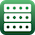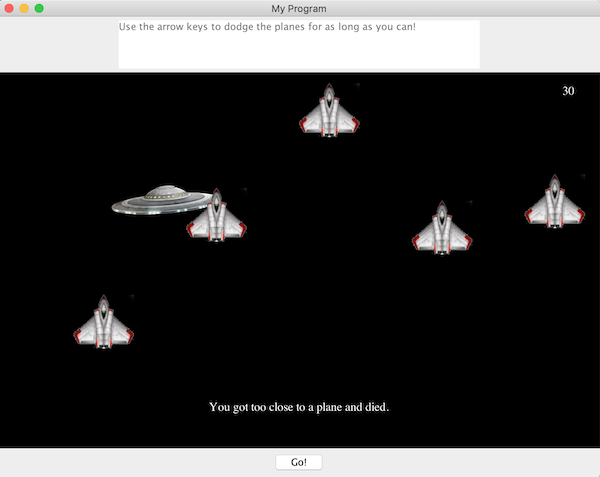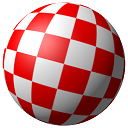Some of the exercises below provide sample runs to better clarify what the program in question is supposed to do. In these sample runs, text given in green has been typed by the user, while white text has been output by the program. Additionally, the "\$" symbol indicates the command prompt, while the "↵" symbol indicates the user has pressed the return key.

Exercises identified with a breadboard logo(shown at left) require the following library:

1.Make a class named SpinningOval that extends the GOval class of the breadboard library and offers an update() instance method that modifies the oval drawn so that it appears to have turned by some number of degrees. In this way, the update() could be called repeatedly using a timer to create an animated effect of the oval spinning along its vertical axis. Create a class called SpinningOvalTest to demonstrate the nature of the SpinningOval class.

import java.awt.Color;

public class SpinningOval extends GOval {

double cx;
double cy;
double theta;
double degreesTurnedPerTick;

public SpinningOval(double x, double y, double radius) {
this.setFilled(true);
this.cx = x;
this.cy = y;
this.theta = 0;
this.degreesTurnedPerTick = 1;
this.setFillColor(Color.YELLOW);
}

public void setTheta(double theta) {
this.theta = theta;
}

public void setDegreesTurnedPerTick(double degrees) {
this.degreesTurnedPerTick = degrees;
}

private double currentWidth() {
return this.getHeight()*Math.abs(Math.cos(theta));
}

private boolean frontFaceShowing() {
return (-Math.PI/2 < theta && theta < Math.PI/2);
}

public double centerX() {
return cx;
}

public double centerY() {
return cy;
}

public void update() {
theta += degreesTurnedPerTick * Math.PI / 180;
this.setSize(this.currentWidth(),this.getHeight());
this.setLocation(this.cx, this.cy);
this.setFillColor( this.frontFaceShowing() ?
Color.YELLOW : Color.ORANGE);
if (theta > 3*Math.PI/2) {
theta = -Math.PI/2;
}
}

public void setLocation(double x, double y) {
this.cx = x;
this.cy = y;
super.setLocation(this.cx - this.currentWidth()/2,
this.cy - this.getHeight()/2);
}

public void move(double dx, double dy) {
this.cx += dx;
this.cy += dy;
this.setLocation(cx+dx,cy+dy);
}
}

import java.util.Random;

public class SpinningOvalTest extends Breadboard {

SpinningOval[][] coins;
GOval oval;

public static void main(String[] args) {
new SpinningOvalTest();
}

public SpinningOvalTest() {
System.out.println("started..");
Random random = new Random();

coins = new SpinningOval;
for (int i = 0; i < 5; i++) {
for (int j = 0; j < 5; j++) {
coins[i][j] = new SpinningOval(150+50*i,100+50*j,40);
coins[i][j].setTheta(2*Math.PI * random.nextDouble());
coins[i][j].setDegreesTurnedPerTick(1 + random.nextInt(3));
}
}

this.getTimer().setDelay(5);
this.getTimer().start();

SpinningOval c = new SpinningOval(50,30,50);
c.setDegreesTurnedPerTick(3);
oval = c;

}

public void onTimerTick() {
for (int i = 0; i < 5; i++) {
for (int j = 0; j < 5; j++) {
coins[i][j].update();
}
}

oval.move(1,0);
((SpinningOval) oval).update();

if (oval.getLocation().getX() > 500) {
oval.setLocation(-50, 30);
}
}
}

2.Write a class named Space that extends the Breadboard class where one guides an alien spaceship through a series of ascending planes.

The spaceship should initially appear in the top left corner of the screen and be controlled by the user through the use of the arrow keys. A single tap of any arrow key should start the ufo moving in that direction, continuing until the edge of the window is reached or a different arrow key is pressed.

Meanwhile, five planes equally spaced across the width of the screen and moving at random but constant speeds should rise from the bottom of the screen until they disappear at the top -- only to reappear again from the bottom again.

If the ufo gets too close to a plane, it should die -- and an appropriate message should be displayed towards the bottom of the drawing area of the window.

The instructions to the user should simply be: "Use the arrow keys to dodge the planes for as long as you can!" and a counter (representing your score) shown in the top right corner of the drawing area should increase as long as the ufo doesn't die by getting too close to a plane. When the ufo dies, this counter should stop increasing.Hints:

• Use the following pictures (both PNG files with transparency) and a GCompound to create the Plane and Ufo classes.import java.awt.Color;
import java.awt.Rectangle;
import java.util.Random;

public class SpaceGame extends Breadboard {

public static final int BREAD_BOARD_HEIGHT = 500;
public static final int BREAD_BOARD_WIDTH = 800;

Ufo ufo;
Plane[] planes;
boolean gameOver = false;
GLabel scoreLabel;

public static void main(String[] args) {
new SpaceGame();
}

public SpaceGame() {

this.getButton1().setText("Go!");
this.getTextArea().setText("Use the arrow keys to dodge the planes for as long as you can!");

//remove some unwanted components...
this.getPanel(this.SOUTH).remove(this.getLabel());
this.getPanel(this.SOUTH).remove(this.getTextField());
this.getPanel(this.SOUTH).remove(this.getButton2());
this.pack();

this.setBackground(Color.BLACK);
}

public void onButton1Click() {
scoreLabel = new GLabel("0");
scoreLabel.setFontSize(16);
scoreLabel.setColor(Color.WHITE);
scoreLabel.setLocation(750,30);

ufo = new Ufo(new Rectangle(800,500));
ufo.setDelay(10);
ufo.setStep(2);

Random random = new Random();
planes = new Plane;
for (int i = 0; i < 5; i++) {
planes[i].setVelocity(0, -2*random.nextDouble());
}
for (int i = 0; i < 5; i++) {
}

//set the breadboard up to respond to key presses
this.setFocusable(true);
this.requestFocusInWindow();

this.getTimer().start();
}

public void onTimerTick() {
for (int i = 0; i < 5; i++) {
if (ufo.getBounds().intersects(planes[i].getBounds())) {
this.gameOver = true;
}
}
if (! this.gameOver) {
this.scoreLabel.setText("" + (1+Integer.parseInt(this.scoreLabel.getText())));
}
else {
//this.setBackground(Color.RED);
this.deadLabel.setText("You got too close to a plane and died.");
}
}
}

import java.awt.Rectangle;
import java.awt.event.ActionEvent;
import java.awt.event.ActionListener;
import java.awt.event.KeyEvent;
import java.awt.event.KeyListener;

import javax.swing.Timer;

public class KeyControlledGCompound extends GCompound {

public static final int UP = 38;
public static final int RIGHT = 39;
public static final int DOWN = 40;
public static final int LEFT = 37;
public static final int STOPPED = -1;

private int direction;
private KeyListener keyListener;
public Timer timer;
private int delay = 10;
private int step = 1;
private Rectangle bounds;

public KeyListener getKeyListener() {
return keyListener;
}

public int getDelay() {
return delay;
}

public int getStep() {
return step;
}

public void setDelay(int delay) {
this.delay = delay;
}

public void setStep(int step) {
this.step = step;
}

public void setBounds(int minX, int maxX, int minY, int maxY) {
bounds = new Rectangle(maxX-minX, maxY-minY);
bounds.setLocation(minX, minY);
}

public boolean inBounds() {
return (bounds.contains(this.getX(), this.getY()) &&
bounds.contains(this.getX() + this.getWidth(),
this.getY() + this.getHeight()));
}

public KeyControlledGCompound(Rectangle bounds) {
this.bounds = bounds;

keyListener = new KeyListener() {

public void keyPressed(KeyEvent e) {
direction = e.getKeyCode();
KeyControlledGCompound.this.timer.start();
}

public void keyReleased(KeyEvent arg0) {}
public void keyTyped(KeyEvent arg0) {}};

timer = new Timer(delay, new ActionListener() {

public void actionPerformed(ActionEvent e) {
KeyControlledGCompound.this.timer.start();
switch (direction) {
case LEFT :
KeyControlledGCompound.this.move(-step,0);
if (! inBounds()) {
KeyControlledGCompound.this.move(step,0);
}
break;
case UP :
KeyControlledGCompound.this.move(0,-step);
if (! inBounds()) {
KeyControlledGCompound.this.move(0,step);
}
break;
case RIGHT :
KeyControlledGCompound.this.move(step,0);
if (! inBounds()) {
KeyControlledGCompound.this.move(-step,0);
}
break;
case DOWN :
KeyControlledGCompound.this.move(0,step);
if (! inBounds()) {
KeyControlledGCompound.this.move(0,-step);
}
break;
case STOPPED :
break;
}
}});
}
}

import java.awt.Rectangle;

import javax.swing.Timer;

public class Ufo extends KeyControlledGCompound {

GImage ufoImage;
Timer timer;

public Ufo(Rectangle bounds) {
super(bounds);
ufoImage = GImage.getFromResource("spacegame/ufo.png",Ufo.class);
}

}

import java.awt.event.ActionEvent;
import java.awt.event.ActionListener;
import java.util.Random;

import javax.swing.Timer;

public class Plane extends GCompound {

private static Random random = new Random();

private GImage planeImage;
private Timer timer;
private double vx;
private double vy;

public Plane(double x, double y) {
planeImage = GImage.getFromResource("spacegame/plane.png", Plane.class);
this.setLocation(x, y);

timer = new Timer(10,new ActionListener() {

@Override
public void actionPerformed(ActionEvent e) {
Plane.this.move(vx,vy);
if (getY() < -planeImage.getImage().getHeight()) {
getHeight());
}
}});

timer.setDelay(20);
timer.start();
}

public void setVelocity(double vx, double vy) {
this.vx = vx;
this.vy = vy;
}

}

3.Create a class named DraggableGOval that extends the GOval class in such a way that once an instance of this class has been added to the canvas, this instance can repeatedly be dragged with the mouse to some new location.

The creation and use of objects of this type should be virtually identical to that of GRect objects, as the below code suggests:

import java.awt.Color;

public class DraggableGOvalTest extends OneButtonBreadboard {

public void run() {
DraggableGOval oval = new DraggableGOval(100,100);
oval.setLocation(200,200);
oval.setFilled(true);
oval.setFillColor(Color.RED);
}
}

import java.awt.event.MouseEvent;
import java.awt.event.MouseListener;
import java.awt.event.MouseMotionListener;

public class DraggableGOval extends GOval {

// instance variable representing the difference in x-coordinates between
// where this object was clicked and where it's top left corner is..
private double xOffset;

// instance variable representing the difference in y-coordinates between
// where this object was clicked and where it's top left corner is..
private double yOffset;

public DraggableGOval(double width, double height) {
super(width, height);

public void mouseClicked(MouseEvent e) {}

public void mousePressed(MouseEvent e) {
if (DraggableGOval.this.contains(e.getX(),e.getY())) {
DraggableGOval.this.xOffset = e.getX()-DraggableGOval.this.getX();
DraggableGOval.this.yOffset = e.getY()-DraggableGOval.this.getY();
}
}

public void mouseReleased(MouseEvent e) {}
public void mouseEntered(MouseEvent e) {}
public void mouseExited(MouseEvent e) {}
});

@Override
public void mouseDragged(MouseEvent e) {
double newX = e.getX()-DraggableGOval.this.xOffset;
double newY = e.getY()-DraggableGOval.this.yOffset;
DraggableGOval.this.setLocation(newX, newY);
}

@Override
public void mouseMoved(MouseEvent e) {}
});
}
}

4.Write a class named SpinningBall that extends GCompound that when added to any Breadboard immediately shows itself spinning with no additional code. The following file provides the individual frames of the ball that should be used to accomplish the animation: ball_frames.zipThe following code can be used to test the SpinningBall class. Upon running this code you should see the ball at right, spinning.

public class SpinningBallFun extends Breadboard {

public static void main(String[] args) {
new SpinningBallFun();
}

public SpinningBallFun() {
this.getPanel(this.NORTH).remove(this.getTextArea());
this.getPanel(this.SOUTH).remove(this.getLabel());
this.getPanel(this.SOUTH).remove(this.getTextField());
this.getPanel(this.SOUTH).remove(this.getButton2());
this.getButton1().setText("Spin!");
}

public void onButton1Click() {
SpinningBall spinningBall = new SpinningBall();
spinningBall.setLocation(110,80);
}
}

package spinning;

import java.awt.event.ActionEvent;
import java.awt.event.ActionListener;
import java.awt.image.BufferedImage;
import java.io.IOException;
import java.io.InputStream;

import javax.imageio.ImageIO;
import javax.swing.Timer;

public class SpinningBall extends GCompound {

private final int numFrames = 59;
private final int timerDelay = 50;

private int currentFrame;
private GImage[] frames;
private Timer timer;

public SpinningBall() {
currentFrame = 0;

frames = new GImage[numFrames];
for (int i = 0; i < numFrames; i++) {
frames[i] = GImage.getFromResource("spinning/bframes/" + frameName(i+1),SpinningBall.class);
}

timer = new Timer(timerDelay, new ActionListener() {
public void actionPerformed(ActionEvent arg0) {
}});
timer.start();
}

this.remove(frames[currentFrame]);
currentFrame = (currentFrame + 1) % numFrames;
}

private String frameName(int i) {
// assuming no more than 9999 frames, returns
// a string representing a filename of the form
// ####.png where the digits preceding ".png"
// are determined by the value of i, with leading
// zeros (examples: 0001.png, 0043.png, 7302.png)

if (i < 10) return "000" + i + ".png";
else if (i < 100) return "00" + i + ".png";
else if (i < 1000) return "0" + i + ".png";
else return i + ".png";
}
}

5.Write classes named ShootingRange, Pidgeon, and Bullet in a package named skeetshooter, so that ShootingRange extends Breadboard

When ShootingRange is run the result should be a simulation of a shooting range, where one fires a rifle at clay pigeons periodically launched up into the air.

The up-arrow key on the keyboard should launch a clay pigeon, while the spacebar should fire a bullet from the rifle. Only one bullet and/or pigeon should be on the screen at any one time, so if the user strikes either key before its related element has left the screen, that key event should be ignored. When the pigeon is launched, it should travel in a parabolic arc, traveling left, over the rifle. The rifle should fire at a slight leftward-pointing angle.

The rifle should be in a fixed position, so success in hitting the pigeon with the bullet will be a matter of timing, not aiming.

Both the pigeon and the bullet should get slightly smaller as they remain in the air, to give the illusion that they are getting farther away (although this effect will be more noticeable with the pigeon, than it will with the bullet, as the bullet already starts out fairly small).

The images linked to below can be used for the in-game graphics.

package skeetshooter;

import java.awt.Color;
import java.awt.event.ActionEvent;
import java.awt.event.ActionListener;
import java.awt.event.KeyEvent;
import java.awt.event.KeyListener;

import javax.swing.Timer;

public class ShootingRange extends Breadboard {

private final int TIMER_DELAY = 2;
private final int SPACEBAR = 32;
private final int UP_ARROW = 38;
private final int WINDOW_WIDTH = 800;
private final int WINDOW_HEIGHT = 500;

private Pidgeon pigeon;
private Bullet bullet;
private Timer timer;
private GImage rifleImage;
private GRect sky;

public static void main(String[] args) {
new ShootingRange();
}

public ShootingRange() {
this.getTextArea().setText("Up arrow launches a pigeon. Space bar fires the gun.");
this.setCenterPanelSize(WINDOW_WIDTH,WINDOW_HEIGHT);
public void keyPressed(KeyEvent e) {
switch (e.getKeyCode()) {
case SPACEBAR : if (bullet == null)
shoot();
break;
case UP_ARROW : if (pigeon == null)
launchPidgeon();
break;
}
}
public void keyReleased(KeyEvent e) {}
public void keyTyped(KeyEvent e) {}
});
this.requestFocus();
}

public void init() {
sky = new GRect(WINDOW_WIDTH, WINDOW_HEIGHT);
sky.setFilled(true);
sky.setFillColor(new Color(220,220,255));

rifleImage = GImage.getFromResource("skeetshooter/rifle.png",ShootingRange.class);
//rifleImage = new GImage("rifle.png");
rifleImage.setLocation(WINDOW_WIDTH/2,
WINDOW_HEIGHT
- rifleImage.getHeight()
);

timer = new Timer(TIMER_DELAY, new ActionListener() {
public void actionPerformed(ActionEvent e) {
if (pigeon != null) {
pigeon.movePerTick();
if (pigeon.getY() > WINDOW_HEIGHT) {
ShootingRange.this.remove(pigeon);
pigeon = null;
}
}
if (bullet != null) {
bullet.movePerTick();
if (bullet.getY() < 0) {
ShootingRange.this.remove(bullet);
bullet = null;
}
}
if ((bullet != null) && (pigeon != null)) {
//System.out.println("bulletBounds = " + bullet.getBounds());
//System.out.println("pigeonBounds = " + pigeon.getBounds());
if (bullet.getBounds().intersects(
pigeon.getBounds())) {

pigeon.explode();
}
}
}});
timer.start();
}

public void launchPidgeon() {
pigeon = new Pidgeon(WINDOW_WIDTH,WINDOW_HEIGHT - 30);
pigeon.setVelocity(-2, -2.9);
}

public void shoot() {
bullet = new Bullet(WINDOW_WIDTH / 2,
WINDOW_HEIGHT - rifleImage.getHeight());
bullet.setVelocity(-2.6, -6);
}

}

package skeetshooter;

import java.awt.Color;

public class Pidgeon extends GCompound {

private final double PIDGEON_WIDTH = 40;
private final double SHRINKAGE_RATE = 0.997;

private GOval bottom;
private GOval top;
private GImage explosion;
private double xVelocity;
private double yVelocity;
private double yAcceleration = 0.01;

public Pidgeon(double x, double y) {
this.bottom = new GOval(0,0);
this.bottom.setFilled(true);
this.bottom.setFillColor(Color.RED);

this.top = new GOval(0,0);
this.top.setFilled(true);
this.top.setFillColor(Color.ORANGE);

this.setWidth(PIDGEON_WIDTH);
this.setLocation(x,y);

this.explosion = GImage.getFromResource("skeetshooter/explosion.png",ShootingRange.class);
//this.explosion = new GImage("explosion.png");
this.explosion.setLocation(-explosion.getWidth()/2,
-explosion.getHeight()/2);
}

public double getWidth() {
return bottom.getWidth();
}

public void setWidth(double width) {
this.bottom.setSize(width,width/2);
this.top.setSize(width,width/2);
this.bottom.setLocation(-width/2,-width/6+width/20);
this.top.setLocation(-width/2,-width/6-width/20);
}

public void setVelocity(double vx, double vy) {
this.xVelocity = vx;
this.yVelocity = vy;
}

public void explode() {
}

public void movePerTick() {
this.move(xVelocity, yVelocity);
this.yVelocity += yAcceleration;
this.setWidth(SHRINKAGE_RATE * this.getWidth());
}

}

package skeetshooter;

import java.awt.Color;

public class Bullet extends GCompound {

private final double ACCELERATION_DUE_TO_GRAVITY = 0.01;
private double SHRINKAGE_RATE = 0.995;
private int DIAMETER= 10;

private double xVelocity;
private double yVelocity;

private GOval oval;

public Bullet(double x, double y) {
this.oval = new GOval(50,50);
this.oval.setFilled(true);
this.oval.setFillColor(Color.BLACK);
this.setDiameter(DIAMETER);
this.setLocation(x,y);
}

public double getDiameter() {
return oval.getWidth();
}

public void setDiameter(double diameter) {
this.oval.setSize(diameter,diameter);
this.oval.setLocation(-diameter/2,-diameter/2);
}

public void setVelocity(double vx, double vy) {
this.xVelocity = vx;
this.yVelocity = vy;
}

public void movePerTick() {
this.move(xVelocity, yVelocity);
this.yVelocity += ACCELERATION_DUE_TO_GRAVITY;
this.setDiameter(SHRINKAGE_RATE * this.getDiameter());
}
}